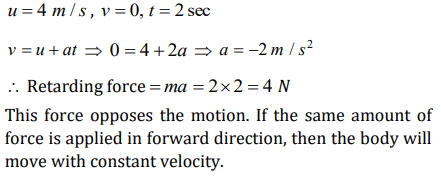## Laws of Motion Questions and Answers Part-2

1. A diwali rocket is ejecting 0.05 kg of gases per second at a velocity of 400 m/sec. The accelerating force on the rocket is
a) 20 dynes
b) 20 N
c) 22 dynes
d) 1000 N

Explanation:2. A body of mass 2 kg moving on a horizontal surface with an initial velocity of 4 m/sec comes to rest after 2 sec. If one wants to keep this body moving on the same surface with a velocity of 4 m/sec, the force required is
a) 8 N
b) 4 N
c) Zero
d) 2 N

Explanation:3. A body of mass 2 kg is hung on a spring balance mounted vertically in a lift. If the lift descends with an acceleration equal to the acceleration due to gravity ‘g’, the reading on the spring balance will be
a) 2 kg
b) $\left(4\times g\right) kg$
c) $\left(2\times g\right) kg$
d) Zero

Explanation: Reading on the spring balance = m (g – a)
and since a = g
Force = 0

4. In the above problem, if the lift moves up with a constant velocity of 2 m/sec, the reading on the balance will be
a) 2 kg
b) 4 kg
c) Zero
d) 1 kg

Explanation: The lift is not accelerated, hence the reading of the balance will be equal to the true weight.
R = mg = 2g Newton or 2 kg

5. In the above problem if the lift moves up with an acceleration equal to the acceleration due to gravity, the reading on the spring balance will be
a) 2 kg
b) $\left(2\times g\right) kg$
c) $\left(4\times g\right) kg$
d) 4 kg

Explanation: When lift moves upward then reading of the spring balance,
R = m(g + a) = 2(g + g) = 4g N = 4 kg [As a = g ]

6. A coin is dropped in a lift. It takes time $t_{1}$to reach the floor when lift is stationary. It takes time $t_{2}$ when lift is moving up with constant acceleration. Then
a) $t_{1}>t_{2}$
b) $t_{2}>t_{1}$
c) $t_{1}=t_{2}$
d) $t_{1}>>t_{2}$

Explanation:7. If the tension in the cable of 1000 kg elevator is 1000 kg weight, the elevator
a) Is accelerating upwards
b) Is accelerating downwards
c) May be at rest or accelerating
d) May be at rest or in uniform motion

Explanation: Since T= mg, it implies that elevator may be at rest or in uniform motion

8. A man weighing 80 kg is standing in a trolley weighing 320 kg. The trolley is resting on frictionless horizontal rails. If the man starts walking on the trolley with a speed of 1 m / s, then after 4 sec his displacement relative to the ground will be
a) 5 m
b) 4.8 m
c) 3.2 m
d) 3.0 m

Explanation:9. In doubling the mass and acceleration of the mass, the force acting on the mass with respect to the previous value
a) Decreases to half
b) Remains unchanged
c) Increases two times
d) Increases four times

10. A force of 5 N acts on a body of weight 9.8 N. What is the acceleration produced in $m\diagup sec^{2}$